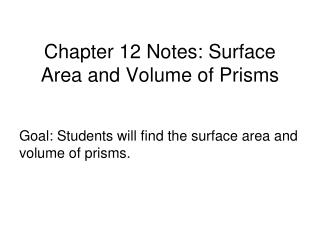DownloadDownload PresentationChapter 12 Notes: Surface Area and Volume of Prisms

# Chapter 12 Notes: Surface Area and Volume of Prisms

Télécharger la présentation## Chapter 12 Notes: Surface Area and Volume of Prisms

- - - - - - - - - - - - - - - - - - - - - - - - - - - E N D - - - - - - - - - - - - - - - - - - - - - - - - - - -
##### Presentation Transcript

1. Chapter 12 Notes: Surface Area and Volume of Prisms Goal: Students will find the surface area and volume of prisms.

2. A prism is a polyhedron with two congruent faces, called bases, that lie in parallel planes. The other faces, called lateral faces, are parallelograms formed by connecting the corresponding vertices of the bases. The segments connecting these vertices are lateral edges. Prisms are classified by the shapes of their bases.

3. Right Prisms: The height of a prism is the perpendicular distance between its bases, called an altitude. A prism may be either right or oblique. In a right prism, each lateral edge is perpendicular to both bases. A prism with lateral edges that are not perpendicular to the bases is an oblique prism.

4. Theorem 12.2 Surface Area of a Right Prism: • The lateral area of a prism is the sum of the areas of the lateral faces. • The surface area of a prism is the sum of the areas of the lateral faces and the two bases. Lateral Area: L.A = ph Surface Area: S = ph + 2B where P is the perimeter of the base, h is the height of the prism, and B is the area of the base.

5. Ex.1: Find (a) the lateral area, and (b) the surface area of the prism. 3 cm 4 cm 6 cm

6. Ex.2: Find the lateral area and surface area of a right rectangular prism with height 7 inches, length 3 inches, and width 4 inches. Ex.3: Find the surface area of the right pentagonal prism.

7. Volume of Prisms Postulate 27 Volume of a Cube: V = s3 where s is the length of the base edge. Ex.4: Find the volume of a cube that has a side length of 6 cm.

8. Theorem 12.6 Volume of a Prism: Volume: V = Bh where B is the area of the base, and h is the height of the prism.

9. Ex.6: 10 cm 20 cm 24 cm

10. Ex.7: 10 in 8 in 8 in 8 in

11. Ex.8: The volume of a triangular prism is 1860 cm3. Its base is a right triangle with legs 24 cm and 10 cm long. a. Draw and label a diagram. b. Find the area of the base of the prism. c. Find the height of the prism.

12. Ex.10: Find the volume of the right hexagonal prism.

13. Ex.11: Find the volume of the puzzle piece in cubic units.

14. Ex.12: Find the surface area of the solid formed by the net. Round your answer to two decimal places.